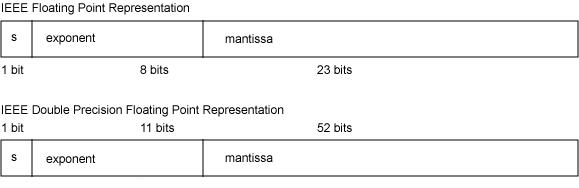# 1.浮点数的存储格式

IEEE754 标准中规定 float 单精度浮点数在机器中表示用 1 位表示数字的符号，用 8 位表示指数，用 23 位表示尾数，即小数部分。对于 double 双精度浮点数，用 1 位表示符号，用 11 位表示指数，52 位表示尾数，其中指数域称为阶码。IEEE754 浮点数的格式如下图所示。# 2.移码

（1）原码符号位为1不变，整数的每一位二进制数位求反得反码；
（2）反码符号位为1不变，反码数值位最低位加1得补码。

# 3.浮点数的规格化

## 3.1 单精度浮点数真值

IEEE754 标准中，一个规格化的 32 位浮点数 x 的真值表示为：
x = ( − 1 ) S × ( 1. M ) × 2 e x=(-1)^S\times(1.M)\times2^e
e = E − 127 e=E-127

## 3.2 双精度浮点数真值

64 位的浮点数中符号为 1 位，阶码域为 11 位，尾数域为 52 位，指数偏移值是 1023。因此规格化的 64 位浮点数 x 的真值是：
x = ( − 1 ) S × ( 1. M ) × 2 e x=(-1)^S\times(1.M)\times2^e
e = E − 1023 e=E-1023

# 4.浮点数的具体表示

## 4.1 十进制到机器码

（1）0.5
0.5 = ( 0.1 ) 2 0.5=(0.1)_2 ，符号位S为0，指数为 e = − 1 e=-1 ，规格化后尾数为1.0。

M = [ 000   0000   0000   0000   0000   0000 ] 2 M=[000\ 0000\ 0000\ 0000\ 0000\ 0000]_2

E = [ − 1 ] 移 − 1 = [ 0111   1111 ] 2 − 1 = [ 0111   1110 ] 2 E=[-1]_移-1=[0111\ 1111]_2-1=[0111\ 1110]_2

0.5 = [ 0011   1111   0000   0000   0000   0000   0000   0000 ] 2 0.5=[0011\ 1111\ 0000\ 0000\ 0000\ 0000\ 0000\ 0000]_2

（2）1.5
1.5 = [ 1.1 ] 2 1.5=[1.1]_2 ，符号位为0，指数 e = 0 e=0 ，规格化后尾数为1.1。

M = [ 100   0000   0000   0000   0000   0000 ] 2 M=[100\ 0000\ 0000\ 0000\ 0000\ 0000]_2

E = [ ０ ] 移 − 1 = [ 10000000 ] 2 − 1 = [ 01111111 ] 2 E=_移-1=[1000 0000]_2-1=[0111 1111]_2

1.5 = [ 0011   1111   1100   0000   0000   0000   0000   0000 ] 2 1.5=[0011\ 1111\ 1100\ 0000\ 0000\ 0000\ 0000\ 0000]_2

（3）-12.5
− 12.5 = [ − 1100.1 ] 2 -12.5=[-1100.1]_2 ，符号位S为1，指数e为3，规格化后尾数为1.1001，

M = [ 100   1000   0000   0000   0000   0000 ] 2 M=[100\ 1000\ 0000\ 0000\ 0000\ 0000]_2

E = [ 3 ] 移 − 1 = [ 1000   0011 ] 2 − 1 = [ 1000   0010 ] 2 E=_移-1=[1000\ 0011]_2-1=[1000\ 0010]_2

− 12.5 = [ 1100   0001   0100   1000   0000   0000   0000   0000 ] 2 -12.5=[1100\ 0001\ 0100\ 1000\ 0000\ 0000\ 0000\ 0000]_2

#include <iostream>
using namespace std;

int main() {
float a=0.5;
float b=1.5;
float c=-12.5;

unsigned int* pa=NULL;
pa=(unsigned int*)&a;
unsigned int* pb=NULL;
pb=(unsigned int*)&b;
unsigned int* pc=NULL;
pc=(unsigned int*)&c;

cout<<hex<<"a=0x"<<*pa<<endl;
cout<<hex<<"b=0x"<<*pb<<endl;
cout<<hex<<"c=0x"<<*pc<<endl;

return 0;
}


a=0x3f000000
b=0x3fc00000
c=0xc1480000


## 4.2 机器码到十进制

（1）若浮点数 x 的 IEEE754 标准存储格式为 0x41360000，那么其浮点数的十进制数值的推演过程如下：

0 x 41360000 = [ 0   10000010   011   0110   0000   0000   0000   0000 ] 0x41360000=[0\ 10000010\ 011\ 0110\ 0000\ 0000\ 0000\ 0000]

x = ( − 1 ) S × 1. M × 2 e = + ( 1.011011 ) × 2 3 = + 1011.011 = ( 11.375 ) 10 x=(-1)^S\times1.M\times2^e=+(1.011011)\times2^3=+1011.011=(11.375)_{10}

#include <iostream>
using namespace std;

int main() {
unsigned int hex=0x41360000;
float* fp=(float*)&hex;
cout<<"x="<<*fp<<endl;
return 0;
}


x=11.375


# 5.浮点数的几种特殊情况

（1）0 的表示

-0 的机器码为：1 00000000 000 0000 0000 0000 0000 0000。

x = ( − 1 ) S × ( 1. M ) × 2 e x=(-1)^S\times(1.M)\times2^e
e = E − 127 e=E-127

（2） + ∞ +\infty − ∞ -\infty 的表示

（3）NaN（Not a Number）

# 6.浮点数的精度和数值范围

## 6.1 浮点数的数值范围

（1）最大正数

P o s M a x = ( − 1 ) S × 1. M × 2 e = + ( 1.11111111111111111111111 ) × 2 127 ≈ 3.402823 e + 38 PosMax=(-1)^S\times1.M\times2^e=+(1.111 1111 1111 1111 1111 1111)\times2^{127}\approx3.402823e+38

（2）最小正数

P o s M i n = ( − 1 ) S × 1. M × 2 e = + ( 1.0 ) × 2 − 126 ≈ 1.175494 e − 38 PosMin=(-1)^S\times1.M\times2^e=+(1.0)\times2^{-126} \approx1.175494e-38

（3）最大负数

N e g M a x = ( − 1 ) S × 1. M × 2 e = − ( 1.0 ) × 2 − 126 ≈ − 1.175494 e − 38 NegMax=(-1)^S\times1.M\times2^e=-(1.0)\times2^{-126} \approx-1.175494e-38

（4）最小负数

N e g M i n = ( − 1 ) S × 1. M × 2 e = + ( 1.11111111111111111111111 ) × 2 127 = − 3.402823 e + 38 NegMin=(-1)^S\times1.M\times2^e=+(1.111 1111 1111 1111 1111 1111)\times2^{127}=-3.402823e+38

## 6.2 浮点数的精度

64 位双精度浮点数的尾数域 52 位，因 2 52 − 1 = 4 , 503 , 599 , 627 , 370 , 495 2^{52}-1=4,503,599,627,370,495 ，所以双精度浮点数的十进制的精度最高为 16 位，绝对保证的为 15 位，所以 double 的十进制的精度为 15~16 位。

# 参考文献

 百度百科.移码
 百度知道.关于IEEE754标准浮点数阶码的移码
 白中英.计算机组成原理第四版[M].科学出版社:P16-30
 维基百科.浮点数

03-019690
03-282万+05-245428
12-262万+
09-299361
11-102万+
07-011万+
04-091万+
01-052万+
10-22727
10-311万+
04-281万+
04-22920
01-051万+
10-082410
01-201929
12-244295
04-051326
04-26203
04-081万+